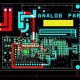# How to Calculate Solar Power for Home Appliances?

In this tutorial, we are going to learn about Calculate Solar Power for Home Appliances.

Here we are taking example of 300W solar system calculation:

Suppose that we have below items in our home for daily uses then we will calculate the power accordingly.

2 Fan which have power of 80W for each then total power will be = 2×80 = 160W

2 Energy saver which have power of 20W for each then total power will be = 2×25 = 50W

1 Led TV which have power of 50W for each then total power will be = 1×50 = 50W

1 Tube Light which have power of 40W for each then total power will be = 1×40 = 40W

Now total power is = 2 Fan 180W +2 Energy Saver 50 W+ 1 Led TV 50W + 1 Tube Light = 300W

For Solar System we need below items:

1. UPS/ Inverter =?
2. Battery = (?)
3. Solar Plates =?

300W solar system calculation: Load = 300W

UPS / Inverter = 500- 1000 W

Power = Voltage X Current

P = VXI

300 = 12XI

I = 300/12 = 25A

Battery Size in AH = W X H / V

Total Load X back up time in hours / Battery Voltage

For 8 Hours back up time

300 X 8 / 12 = 200 AH

For 4 hours back Time

300 X 4 / 12 = 100 AH

Battery Charging current = 1/10TH of batter rating.

= 200/10 = 20A

Solar Plate current = Input Current + Battery Current

Now Solar Plate = 25A + 20 A = 45A

Total Solar Current = 45A

Now we will calculate the solar plate power = V X I

Maximum Solar plate voltage = 14V

Then Solar Plate Power = 45 X 14 = 630W

Solar Plates = 630/150 = 4.2

It means we need total 4 solar plates for this load.